# Defining Methods in JSP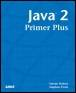Java™ 2 Primer PlusBy Steven Haines, Steve Potts Table of Contents Chapter 22.  JavaServer Pages (JSP)

Many experienced Java programmers want to include modularity in their JSP. One of the best ways to preserve some order is to create methods to perform specific functions. These methods can then be called from within the scriptlet code in the JSP. The value returned can then be displayed. Listing 22.4 shows an example of this.

##### Listing 22.4 The MethodCaller JSP
` <%!      private java.util.Date sinceDate = new java.util.Date();      private java.util.Date getSinceDate()      {          return sinceDate;      }  %>  <html>  <head>  <title>Method Caller</title>  <body>  <h2>The Startup Date</h2>  This page was first run on <%= getSinceDate() %>.  </body>  </html> `

This short example shows some interesting code. The first section starts with a <%!, which tells the JSP engine that everything in here is a declaration. The first thing that we do in this section is create a variable of type Date that will hold the date that this JSP was first run.

` <%!      private java.util.Date sinceDate = new java.util.Date(); `

We could have referred to the sinceDate directly, using an expression, but we use a method call so that you can see how this is done.

`     private java.util.Date getSinceDate()      {          return sinceDate;      }  %> `

The syntax of the call is an expression. Because this method returns the Date object, it is a simple thing to place it inside an expression and display it.

` This page was first run on <%= getSinceDate() %>.  `

The result of running this program is shown here in Figure 22.3:

##### Figure 22.3. The method call is made, and the resulting date is displayed.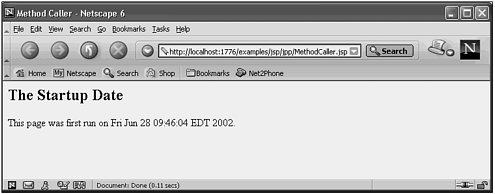Run this same page several times; notice how the time doesn't change. Restart the Tomcat server, and then run this JSP again. Notice now how the date has changed. What we have discovered is that the JSP and the servlet that is generated to run it both remain in memory between calls. This means that all class level servlet variables are static by nature because there is only one instance of the servlet in memory. Whenever a browser runs a page, a new thread of the same servlet is created.

This fact will be clearer after we run the next example. This example shows the classic counter application where the number of hits that the page has received is shown. Listing 22.4 shows this example.

##### Listing 22.5 The MethodCounter JSP
` <%!      private int hits = 1;      private int getHits()      {          return hits++;      }  %>  <html>  <head>  <title>Method Counter</title>  <body>  <h2>The Hit Count</h2>  This page has been accessed <%= getHits() %> times.  </body>  </html> `

The declaration of the variable hits is not stated to be static, but its behavior is as if it were.

` private int hits = 1; `

The method body returns the current value of the counter before incrementing it.

` private int getHits()  {      return hits++;  } `

The result is displayed using an expression:

` This page has been accessed <%= getHits() %> times.  `

The result is shown in Figure 22.4.

##### Figure 22.4. The page counter behaves like a static variable.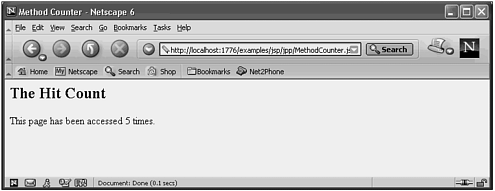Again, if you restart the Web server, the number will reset to one.

#### Conditional Output

One of the most common dynamic HTML activities is to selectively display content, based on some condition. This condition could be tied to the time of day or month of the year, but typically it is tied to a parameter that is passed in. The example shown in Listing 22.6 shows a parameter-based JSP.

##### Listing 22.6 The Temperature JSP
` <html>  <head>  <title>Temperature Translator</title>  <body>  <% float fTemp;     float cTemp;     String sTemp = request.getParameter("temp");     cTemp = Integer.parseInt(sTemp);     fTemp = (cTemp*9/5)+32;  %>  The Fahrenheit Temperature is <%=fTemp %> <br>  <%if (fTemp < 60) %>  <b>It's chilly </b>  <%if (fTemp > 85) %>  <b>It's Hot </b>  <%if ((fTemp >= 60) && (fTemp <= 85)) %>  <b>It's Nice </b>  </body>  </html> `

This example demonstrates how a value can be calculated based on user input, and then that value used to make a conditional choice.

The parameters that are retrieved from a browser are of type String. Before you can do any math with them, you have to convert them to either integer or float values. The Integer class contains a static method called parseInt() that performs this conversion and returns an integer value.

` <% float fTemp;     float cTemp;     String sTemp = request.getParameter("temp");     cTemp = Integer.parseInt(sTemp);     fTemp = (cTemp*9/5)+32;  %> `

After displaying the temperature in the Fahrenheit scale, a conditional test is made to give the user some idea of the quality of the current temperature.

` <%if (fTemp < 60) %>  <b>It's chilly </b>  <%if (fTemp > 85) %>  <b>It's Hot </b>  <%if ((fTemp >= 60) && (fTemp <= 85)) %>  <b>It's Nice </b> `

Notice how the scriptlets start and end on every line. The line following each scriptlet is simple HTML. The conditional statement applies to all lines following the condition until the next scriptlet line is encountered.

The result is shown in Figure 22.5.

##### Figure 22.5. The conditional statements control what is displayed.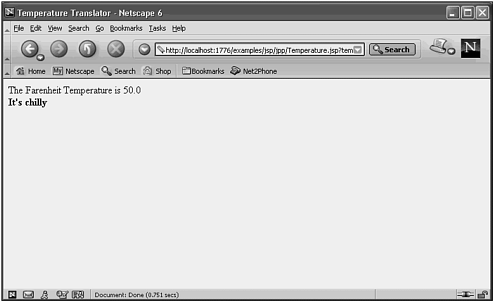#### Calling JSP from HTML Forms

Rather than entering the temperature as part of the URL, it would be more realistic to have the user enter it into an HTML form. Listing 22.7 shows the HTML for this form.

##### Listing 22.7 The Temp.HTML File
` <!DOCTYPE HTML PUBLIC "-//W3C//DTD HTML 4.01 Transitional//EN">  <HTML>    <HEAD>      <TITLE>Passing Parameters to JSPs</TITLE>    </HEAD>    <H1 align="CENTER">    Enter the Temperature in Celcius    </H1>    <FORM action="/examples/jsp/jpp/Temperature.jsp">       Temperature:    <INPUT TYPE="TEXT" NAME="temp"><BR><BR>       <CENTER>          <INPUT TYPE="SUBMIT">       </CENTER>    </FORM>    </BODY>  </HTML> `

JSP references inside the HTML file are identical to the references to servlets, except that the path is normally more logically stated. Servlets are often stored in special directories that don't match the path that you enter on the command line, where JSP references do.

` <FORM action="/examples/jsp/jpp/Temperature.jsp"> `

The HTML form is displayed on the screen, as shown in Figure 22.6.

##### Figure 22.6. The HTML form can call a JSP directly.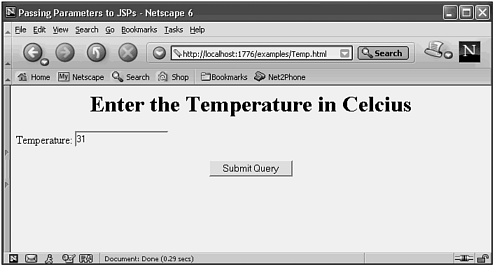#### Error Handling

If the user enters in a letter instead of a number in the Temp.HTML form, an ugly, programmer-oriented error message will result. If Grandma is viewing this page, she will not find much help in resolving her problem. You can include try/catch logic to address this. Listing 22.6 is a modified version of the Temperature.jsp that handles invalid formats.

##### Listing 22.8 The Temperature2.jsp
` <html>  <head>  <title>Temperature Translator</title>  <body>  <%     try     {        float fTemp;        float cTemp;        String sTemp = request.getParameter("temp");        System.out.println("The temp is " + sTemp);        cTemp = Integer.parseInt(sTemp);        fTemp = (cTemp*9/5)+32;  %>  The Fahrenheit Temperature is <%=fTemp %> <br>  <%if (fTemp < 60) %>  <b>It's chilly </b>  <%if (fTemp > 85) %>  <b>It's Hot </b>  <%if ((fTemp >= 60) && (fTemp <= 85)) %>  <b>It's Nice </b>  <%  }   catch (NumberFormatException e)  {  %>  <H2>Invalid Number</H2>  Please make sure that the temperature is a number  <% } %>  </body>  </html> `

The try is placed before the input is retrieved from the request object.

` <%     try     {        float fTemp; `

The catch could have been placed in the same scriptlet, or it could be placed near the end of the JSP as we did here.

` }   catch (NumberFormatException e)  {  %>  <H2>Invalid Number</H2>  Please make sure that the temperature is a number   <% } %>  </body>  </html> `

The result of entering the data incorrectly is a nice error page as shown here in Figure 22.7.

##### Figure 22.7. Try/catch logic can be used in a JSP also.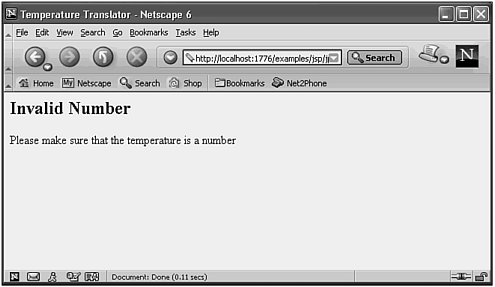This error message is much more likely to provide real information to end users than the stack-trace information that appears by default.

 Top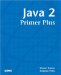Java 2 Primer Plus
ISBN: 0672324156
EAN: 2147483647
Year: 2001
Pages: 332

Similar book on Amazon